QC1 Database User's Guide:
QC1_plotter

QC1 database:
Project
Tech Guide
Users GuideQC1 database: plotter interface

This interface allows to interactively select and filter two keywords from a QC1 database table, and plot the content. It is intended as quick-look tool for visualization, trending analysis and data selection.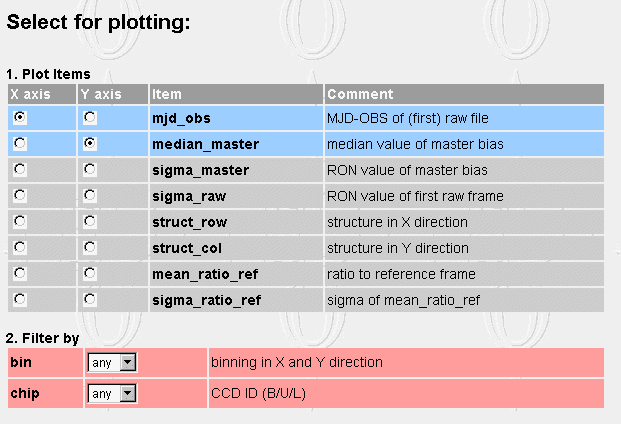Panel 1 is for selecting X and Y axis parameter. Default choices are marked blue. X default is always mjd-obs. The other listed options are the numerical (integer or float) entries of the QC1 table selected.

Panel 2 lists all filter options. These are mostly instrument keywords which can be used to filter the output. E.g., it is generally not useful to plot all mean bias values if there are multiple CCDs and read-out modes.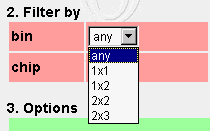All possible (non-NULL) choices which exist in the database are listed, including 'any'. Proper selection may require expert knowledge. E.g., some 20 or so different values for slit width exist for UVES wave calibration frames, and random selection may give useless results.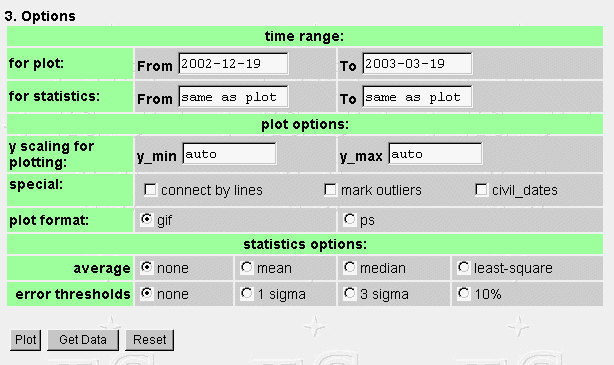Panel 3 has the plot options. You can select:

• time range for data selected to plot: from [default: today minus 3 months] to [default: today]

• time range for statistical analysis: from [same as plot] to [same as plot]
that option is normally left unchanged; it is typically used for trend analysis, if e.g. a mean value for a given period (subset of the plot period) is compared to the whole data set

• y scaling: y_min and y_max can be set. Default is 'auto' and is determined from minima and maxima of the dataset (if no average option is selected), or from the selected average option plus/minus 10%, or from the selected error thresholds plus/minus 10%. The 'auto' choice usually gives reasonable limits.

• special: some options can be clicked here (multiple choices possible):
• connect by lines: all data points in the sample can be connected by lines; useful for checking that no outlier has been lost outside the Y scaling
• mark outliers: statistical outliers are plotted as red asterisks (only possible if statistcs options chosen)
• civil_dates: human-readable civil dates (begin of month) are marked by vertical broken lines; useful for orientation on larger samples

• plot format: can be gif for quick-look, or ps for high-quality postscript prints. In the latter case, the output will be handled according to the preferences set in the browser (e.g. downloaded, shown with ghostview etc.).

• statistics options:
• average: mean, median or linear least-square fits are calculated, plotted and given as numbers
• error thresholds: 1 sigma, 3 sigma or 10% error bars are calculated, plotted and given as numbers.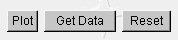After selection, data can be plotted ('plot'). There is also a button to download the selected data set ('Get Data'). This makes sense if the interface has been used to select and plot a sample which is intended for further analysis.

The output has some summary information about the table plotted, the selected keys, time ranges, and filter values.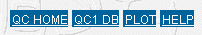The navigation bar on top connects to the QC home page, the top-level (general) QC1 database interface, the corresponding QC1 browser interface, and to this HELP page.

 Output

Let us look at the output of the QC1 plotter. In addition to the above selection, we have filtered 'chip = B' and 'bin = 1x1', but otherwise left all default choices (date fields were default at the time of creating the plot).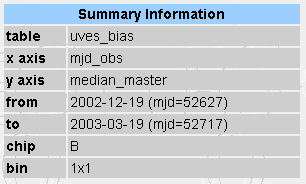The output has a top label field repeating the selected parameters.

This is the graphical output: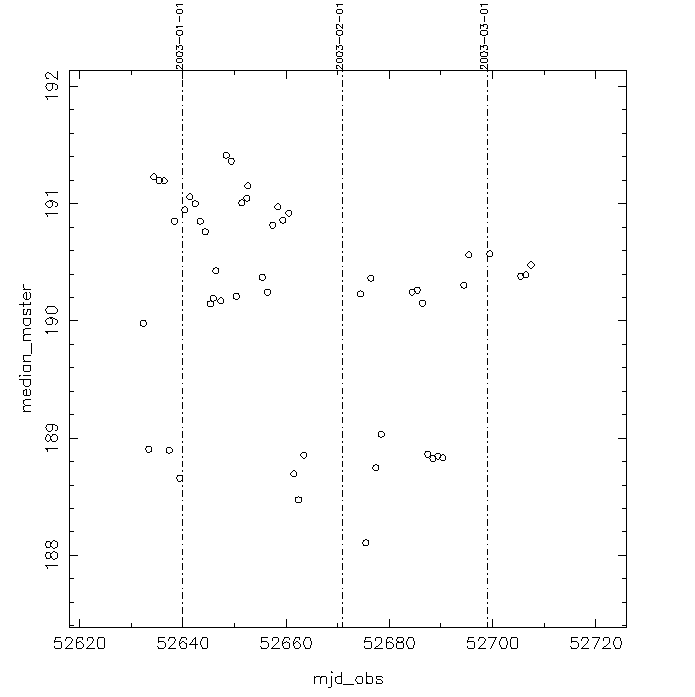It has the mean bias values plotted versus MJD-OBS.

The output can be fine-tuned by setting

• y_min = 160, y_max = 210;
• special: 'mark outliers';
• average: 'mean'
• error thresholds: '1 sigma'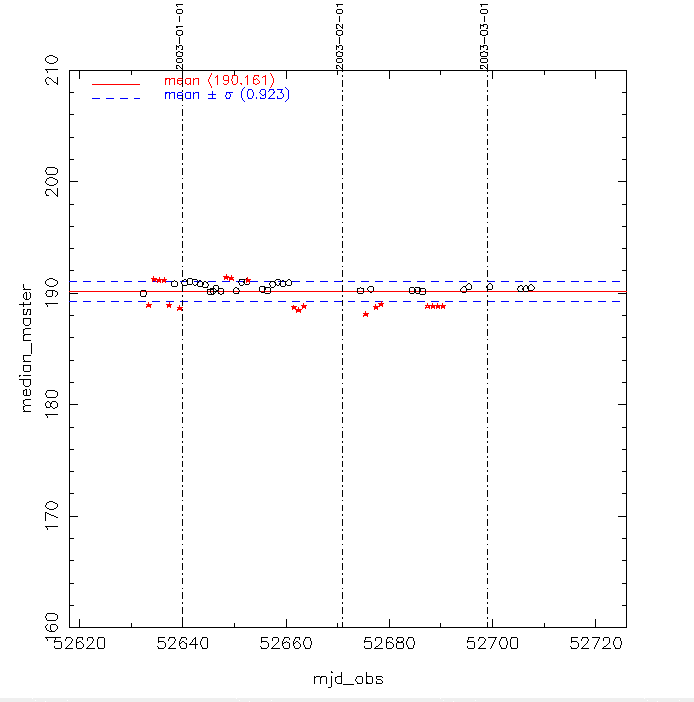This brings the plot to a reasonable scaling. The mean value is given in the plot (190.161) and as red line. The 1-sigma error bar is also indicated (0.923) and plotted as broken blue lines. The 'mark outliers' option turns all those data points into red asterisks which are outside the (mean +/- 1 sigma) range.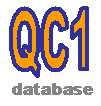Last update: November 25, 2020